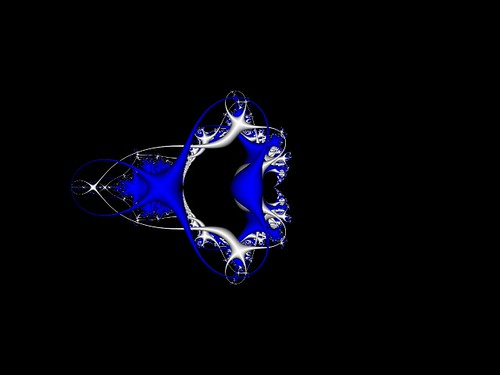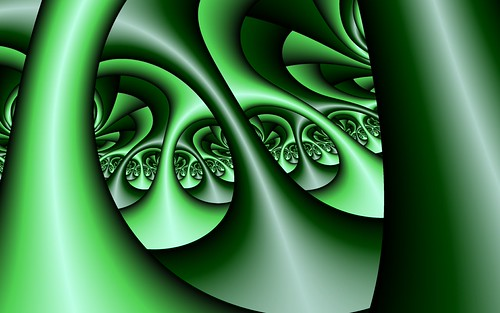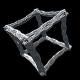# Orbit Traps

In regards to fractals, orbit traps give a new way to render images. As a fractal is created each pixel of the image is calculated by repeating (iterating) a formula over and over. In the case of the Mandelbrot Set the formula is z=z^2+c. As each iteration happens if you follow or plot the path the complex number z takes at each step you can see the “orbit” the point takes.

To see how these orbits behave, open this shader and move your mouse around. The plotted blue lines are the path the iterations for each pixel go through.

The orbit traps themselves are shapes within the complex plane. If the iterating set of points hits this shape then it is “trapped” and the pixel is then colored depending on how close it is to the trap. Not that easy to explain to someone who does not understand the maths involved (and no doubt if you read the above and are not into math then you have a “what the!?” expression now).

Here is the famous Mandelbrot Set.If the “cross” orbit trap is applied you get an image like the following.The main centered white cross shape is the main trap. Any time the orbit of a pixel hits within the cross shape it stops iterating and is shaded based on its location within the trap. If only the trap shape is displayed the result is like this image.There are numerous styles and types of orbit traps out there. Here is another sample of the above Mandelbrot Set rendered with the “stalks” orbit trap.When you zoom into these orbit trap images you get some more interesting results.Source Code

The source code to these orbit traps is included in the Custom Formula Editor of Visions of Chaos. Here are the 5 shaders here for people who want to see how they work.

Root-Finding Fractals Orbit Traps

After expanding the root-finding fractals mode in Visions Of Chaos I have now added the ability to render orbit traps within them. The results are as interesting or more interesting than the Mandelbrot orbit trap images. Here are a few example images.Here is a zoom into the above Newton Fractal using the cross orbit trap and shaded with Perlin Noise.

Even though the movie has a semi 3D appearance of going through a forest of fractal tree trunks the frames are pure 2D. It is the orbit traps that give it the 3D look.

There are many more styles of orbit traps to use and I will no doubt be playing and experimenting with new varieties for years to come.

Jason.

## 7 responses to “Orbit Traps”

1.Benjamin Popp says:

Excellent article and nice examples. I’ve been studying fractals for some time now and I’m just starting to get into orbit traps; you had some good information for beginners. Could you include images or formulas for the basic shapes used for the orbit traps (such as “the cross is .1 units thick/wide, parallel to the x-axis with the intersection at the origin”)? I’m especially interested in the “stalks” shape and the traps on convergent fractals because I haven’t found any good information on it elsewhere on the net. Any pointers would be greatly appreciated.

•softologyblog says:

OK, here is some code that should (?) help.

For the cross.

Initialise the trapsize:=0.05;

Then during each iteration

if (abs(z.x)<trapsize) then
begin
trapdist:=abs(z.x);
trapped:=true;
end
else
if (abs(z.y)<trapsize) then
begin
trapdist:=abs(z.y);
trapped:=true;
end;

z.x and z.y and the complex number real and imaginary components. Once trapped, you stop iterating and shade depending on how close the point was to the trap. ie (trapdist/trapsize*255) for rgb color intensity.

For the stalks trap.

Then during each iteration…

begin
trapped:=true;
end;

Then index the color palette shade by 255-trunc(sqrt(ztot)*256);

Hope that helps you out.

Jason.

•Vasily Makarov says:

Concerning a stalks trap.
What is “stalksradiuslow” and “ztot” variables?
As well as at what stage they are initialized?
May I see the operable code?
Thanks!

•softologyblog says:

Initialise stalksradius:=0.1; This is done once before the main iteration loop.

For each pixel (where you are normally calculating the z=z^2+c) add this code to detect if trapped or not. ztot is intialized to 0 for each pixel before the loop.

begin
trapped:=true;
end;

After the z=z^2+c loop check if trapped is true. If it is trapped then you index the color palette shade by 255-trunc(sqrt(ztot)*256);

Jason.

2.Vasily Makarov says:

Thanks!
However at expression (sqrt (sqr(z.y)+sqr(z.x)) =stalksradiuslow) the trap does not work as the probability of equality of two real numbers in this case it is insignificant is small (in my case the trap never worked).
It was succeeded to achieve operability of an algorithm only on condition that module Z is in the range of stalksradiuslow..stalksradiushigh. That is:

m:=sqrt(sqr(z.y)+sqr(z.x));
begin
trapped:=true;
end;

Where ‘InRange’ is function from the Math unit. The result of work of a trap can be seen here: http://stone-voices.ru/img/ffimg/orbit_trap02.png

There is an opportunity to publish formulas for styles of a trap such as ‘Circles’,’Rings’ and ‘Squares’?

•softologyblog says:

See the updated post above for links to the full shader code for each of the 5 types of orbit traps.

Jason.

3. Pingback: Vines #4 – Spyke:Stage